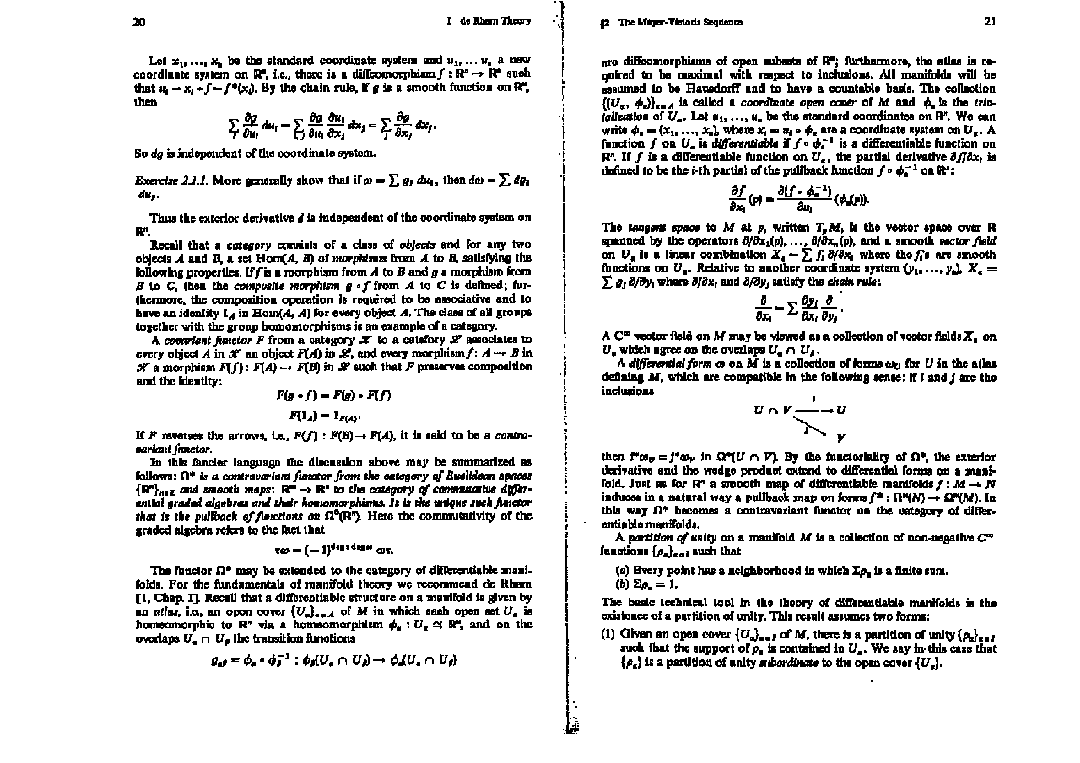# Locally closed subgroups are closed

In a topology group $G$, an open subgroup $H$ is also closed. The proof of this statement is not hard: $G=\bigcup_{g_i} g_iH$ is a disjoint union of open left cosets, where $\{g_i\}$ is a complete representatives set of $G/H$. Then $\bigcup_{g_i \ne 1}g_iH$ is open and $H=G-\bigcup_{g_i \ne 1}g_iH$ is the complement of an open set, and therefore $H$ is closed. In this post, I will prove a slightly more general theorem:

Theorem. Let $G$ be a topological group. If $H$ is a locally closed subgroup in $G$, then $H$ is closed.

We will see that an open subgroup $H$ is locally closed, so it’s closed by the Theorem. Before the proof of the Theorem, let’s talk about locally closed sets first.

Read More

# Examples of split and non-split tori

Let $T$ be an algebraic group. $T$ is called an algebraic torus over $k$, if $T(E)$ is isomorphic to a finite direct product of copies of $G_m(E)$ for some finite finite extension $E$ of $k$, where $G_m$ is the multiplicative group. If $E$ can be $k$, then $T$ is called a split torus over $k$; otherwise, $T$ is called a non split torus over $k$. In this post, I am going to talk something about $SO$ to give examples of non split and split tori.

Definition. Let $k$ be a field, and $V$ be a vector space over $k$. Let $q$ be a quadratic form on $V$. Define $$SO(V,q;k)=\{\gamma \in SL(V) : q(\gamma v)=q(v), \forall v \in V\}.$$

Read More

# The Mellin transform

### Definition

Let $\mathbb{R}^{+}$ be the set of positive real numbers. Given a function $f$ on $\mathbb{R}^{+}$, define the Mellin transform of $f$, whenever it makes sense, as follow: $\mathcal{M}(f)(s)=\int_{0}^{\infty} f(t)t^{s} \frac{dt}{t}. \; (1)$

The very first example of the Mellin transform I have known is the gamma function, $\Gamma(s) = \int_{0}^{\infty} e^{-t}t^{s} \frac{dt}{t},$ which is the Mellin transform of the function $e^{-t}$.

Read More

# $k$ squares problem

Let’s fix a natural number $k$. How many ways can we decompose $n$ into $k$ squares? It’s an interesting problem and it should help me understand automorphic forms better.

Let $w_k(n)$ be the number of tuples $(n_1, \cdots, n_k)\in \mathbb{Z}^k$ such that $n_1^2+\cdots+n_k^2=n$. Let $g_k(q)$ be the generating function of $w_k(n)$, i.e., $g_k(q)=\sum_{n \in \mathbb{N}} w_k(n)q^n.$ Then, $\begin{split} g_k(q) &= \sum_{n \in \mathbb{N}} w_k(n)q^n \\ &= \sum_{n \in \mathbb{N}} \sum_{\substack{n_1, \cdots, n_k \in \mathbb{Z} \\ n_1^2+\cdots+n_k^2=n}}q^n \\ &= \sum_{n_1, \cdots, n_k \in \mathbb{Z}} q^{n_1^2+\cdots+n_k^2} \\ &= \left(\sum_{n \in \mathbb{Z}} q^{n^2}\right)^k \\ &= (g_1(q))^k. \end{split}$

Read More

# Self hypnosis and insomnia

I have been suffering from insomnia since I came back to Columbus. I thought it was just a matter of jet lag. But after a week or two, I began to realize that it is something else, something unknown yet, that matters. Insufficient sleep turns me into a zombie around 2 or 3 pm in the afternoon.

I had exactly the same problem last December. I tried many ways and finally figured out alcohol worked it out for me. However, alcohol has lost its power on me this time, soon after I finished my rum. Now I go to swimming and work out every other day to make me exhausted at the end of day. Theoretically, this should make me tired and then I could fall asleep easier. Reality always beats theory. It just worsen the consequence of insomnia.

Read More

Read More

# 过去的2013

2013年有两件重要的事情：毕业、出国——一个阶段的结束伴随另一个阶段的开始。

Read More

# 冬日碎语

1、前一段时间总是凌晨两三点才能入睡。每当我置身于黑暗中，我就开始想这样的一个问题：上帝是否存在？这个问题的起源是几个“为什么”。为什么物体会往下掉？因为有重力。为什么会有重力？因为有质量的物体能产生重力场，从而产生重力。那为什么有质量的物体能产生重力场？以我现在的知识，我无法解答这个为什么。我觉得无论是怎样的“为什么”，不断追问下去的话，最终总会问到”谁创造宇宙“的问题。

Read More

# 分割双页扫描电子书Read More

Read More